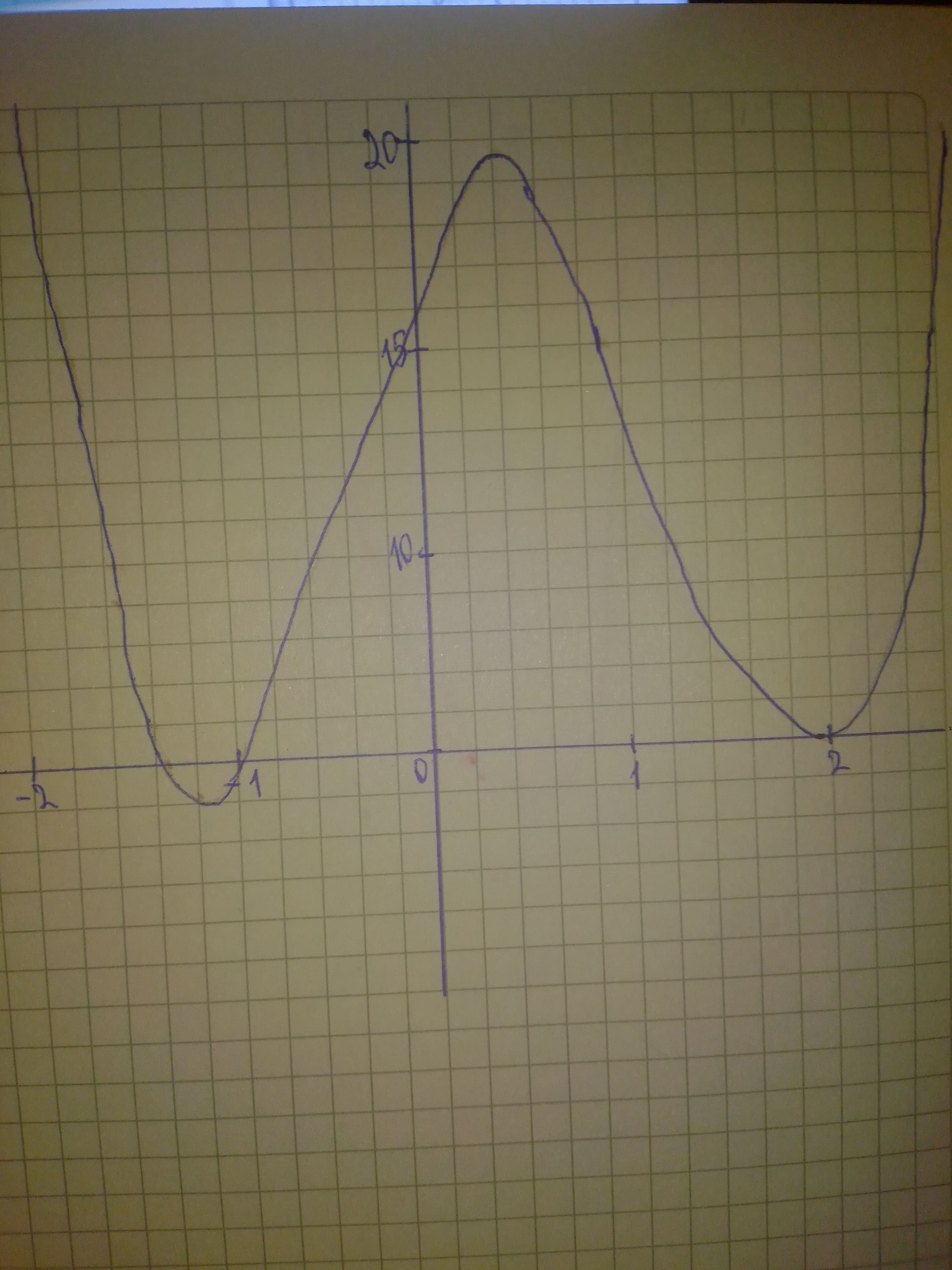# Solve the polynomial inequality graphically. 3x^{4}-5x^{3}-12x^{2}+12x+16Wotzdorfg 2020-10-21 Answered
Solve the polynomial inequality graphically. $3{x}^{4}-5{x}^{3}-12{x}^{2}+12x+16$
You can still ask an expert for help

• Live experts 24/7
• Questions are typically answered in as fast as 30 minutes
• Personalized clear answers

Solve your problem for the price of one coffee

• Math expert for every subject
• Pay only if we can solve itSally Cresswell

Step 1
We graph the left-hand side of the inequality and find x-intercepts at $x=-\frac{4}{3},x=-1,x=2$:We have step-by-step solutions for your answer!